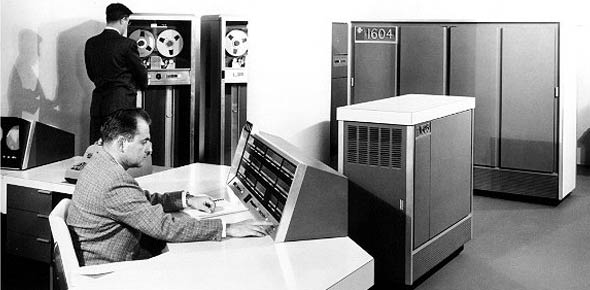# CDC Volume 2

100 Questions | Total Attempts: 152SettingsCreate your own QuizVol 2 2a651 cdc cdc cdc cdc cdc cdc cdc

• 1.
" an unbalanced force on a body produces or tends to produce an acceleration in the direction of the force that the acceleration if any is directly proportional to the force and inversely proportional to the mass of the body." this statement is?
• A.

Bernoulli's principle

• B.

Newton's 1st law of motion

• C.

Newton's 2nd law of motion

• D.

Newton's 3rd law of motion

• 2.
Which type of duct would decrease the velocity and increase the pressure of a gas as it passes through?
• A.

Elbow

• B.

Straight

• C.

Divergent

• D.

Convergent

• 3.
"the combination of decreased pressure above an airfoil and increased pressure below an airfoil produces lift" is best described by?
• A.

Bernoulli's principle

• B.

Newton's 1st law of motion

• C.

Newton's 2nd law of motion

• D.

Newton's 3rd law of motion

• 4.
The tendency of an object at rest to stay at rest and the tendency of an object in motion to remain in motion in a stright line and the same speed is called?
• A.

Energy

• B.

Inertia

• C.

Friction

• D.

Potential

• 5.
Which section of a jet engine introduces and burns fuel?
• A.

Turbine

• B.

Diffuser

• C.

Compressor

• D.

Combustion

• 6.
Which section of a jet engine keeps the compressor rotating?
• A.

Turbine

• B.

Exhaust

• C.

Diffuser

• D.

Combustion

• 7.
Which method of producing thrust does a turbo prop use?
• A.

Accelerating a large mass of air by a small amount

• B.

Moving a small quantity of air through a large velocity change

• C.

Using the same working fluid for propulsive force as that used within the engine

• D.

Using only the energy expended through the exhaust nozzle to propel the aircraft forward

• 8.
Which type of horsepower is delivered to the propeller for useful work?
• A.

Brake

• B.

Indicated

• C.

Frictional

• D.

Equivalent shaft

• 9.
The heat content per pound of kerosene is how many btu's?
• A.

10,500

• B.

15,000

• C.

18,500

• D.

40,000

• 10.
To measure barometric pressure you would use a?
• A.

Potentiometer

• B.

Psychrometer

• C.

Pyrometer

• D.

Barometer

• 11.
What is the sea level atmospheric pressure at "standard day" conditions?
• A.

13.5psi

• B.

14.7psi

• C.

49.94psi

• D.

40.55psi

• 12.
What effect does the divergent design of a diffuser have on air pressure?
• A.

Increases pressure

• B.

Decreases pressure

• C.

Has no effect on pressure

• D.

Results in pressure fluctuations

• 13.
Which engine component meters fuel for combustion?
• A.

P&D valve

• B.

Fuel pump

• C.

Fuel control

• D.

Fuel nozzles

• 14.
What directs the gases onto the first stage turbine wheel blades in a jet engine?
• A.

Flameholder

• B.

Combustion chamber

• C.

• D.

Turbine stator

• 15.
When two or more turbine wheels are used in a jet engine, which component is placed directly in front of each turbine wheel?
• A.

Diffuser

• B.

Jet nozzle

• C.

Combustion chamber

• D.

Turbine stator (nozzle diaphragm)

• 16.
The three major sections of all jet engines are compressor, combustion, and?
• A.

Turbo

• B.

Turbine

• C.

Exhaust

• D.

Diffuser

• 17.
Vane-type fuel pumps used in jet engines are similar to?
• A.

Sliding vane air compressors

• B.

Piston pump air compressors

• C.

Roots air compressors

• D.

Turbo-superchargers

• 18.
The 2 forces air is subjected to after it is drawn into the guide vanes of a centrifugal compressor are?
• A.

Rotational and centrifugal

• B.

Rotational and tangential

• C.

Tangential and centrifugal

• D.

• 19.
A constructional feature of the centrifugal compressor is that the impeller?
• A.

Has 2 matching plates

• B.

Has a lightweight design

• C.

Is forged as a single unit

• D.

Is constructed in rows of blades and stators

• 20.
The forward end of the shaft on a centrifugal compressor is hollow in order to?
• A.

Act as a vibrational damper

• B.

Allow cooling air to the turbine

• C.

Provide a passage for lubricating oil

• D.

Provide a passage for the main fuel supply

• 21.
Stationary vanes positioned between rotor discs in a compressor are used to?
• A.

Direct air and increase pressure

• B.

Direct hot gases rearward

• C.

Increase backup pressure

• D.

Prolong compressor life

• 22.
In a dual spool compressor RPM of the N2 compressor are determined by the?
• A.

Starter

• B.

Fuel pump

• C.

Fuel control

• D.

Low pressure turbine

• 23.
Which facet of engine design helps prevent igniter plug fouling
• A.

Gold plating on the plug

• B.

Split electrode on the plug

• C.

Ceramic coating on the plug

• D.

Directing air around the plug

• 24.
The excess air that is not burned in the combustion section is used to?
• A.

Cool the burner surfaces

• B.

Operate pneumatic accessories

• C.

Decrease the mass of exhaust gases

• D.

Increase air in the compressor section

• 25.
Fuel that accumulates after a failed start is?
• A.

Returned to the fuel control by the tubes

• B.

Drained overboard by the drain system

• C.

Burned on the next start attempt

• D.

Allowed to evaporate

Related TopicsBack to top
×

Wait!
Here's an interesting quiz for you.